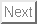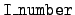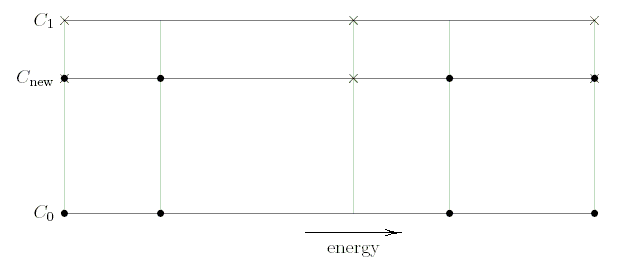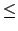Up: Code details for mcfgen Previous: Equiprobable bins   Contents

# Correlated angle-energy equidistribution

The algorithm to produce equidistributed correlated angle-energy bins from= 3 data is implemented by the routine iequ3. The data for both the angle-energy probability densities (= 3) and for the angular probability densities (= 1) must be present. Furthermore, the lists of incident energies at which the= 3 and= 1 probability densities are given must be identical.

The computation of equiprobable angle-energy is done in three steps. First, the= 1 data is used to make an equidistribution of the cosines using the method described above. For each of these equidistributed cosines we need to create equidistributed secondary energy bins. So, the second step is the creation of secondary energy distributions at these new cosines. The third step is the equidistribution of these energies. The only new step here is the second step, that of making an energy distribution at one of the edges of an equiprobable cosine bin. Its details are as follows.

Consider one of the equidistributed cosines. We bracket it between two= 3 (or equivalently,= 1) cosines from the library' file. We want to use the (energy, probability density) data from these bracketing cosines to produce (energy, probability density) pairs for the new cosine. This is illustrated in the Fig. 2, which has secondary energy increasing to the right and cosine increasing upward. Suppose that the new cosine Cnew lies between the library cosines C0 and C1. We want probability densities for Cnew, given probability densities at one set of energies for C0 (denoted by dots) and at another set of energies for C1 (denoted by crosses). The algorithm is based on multiple linear interpolation as follows.Figure 4.2: Double interpolation of probability density.

1.
We start by translating and scaling the secondary energy ranges for the cosines C0 and C1 to the interval 0E1, and we scale the probability densities correspondingly. The figure shows the situation after this scaling. There are several reasons for starting with this scaling. For one thing, it ensures proper linear dependence on incident energy for the minimum and maximum energy of the secondary particle. For another, it leads to more accurate interpolation of such physical features as resonance peaks. Finally, it makes the interpolation process clearer.

2.
We merge these scaled energy lists to produce a master secondary energy list, denoted by the vertical lines in the figure.

3.
For each library cosine C0 and C1 we interpolate in energy to get probability densities at the full merged list of secondary energies. This is interpolation horizontally along the top and bottom of the rectangle in Fig. 2.

4.
Then at each secondary energy we interpolate vertically in the figure, to get the probability densities at the equiprobable cosine  Cnew.

5.
Now that we have probability densities, we use the method described in the previous section to get equiprobable secondary energy bins at  Cnew, but these are still scaled to 0E1.

6.
Finally, we undo the scaling by mapping from 0E1 to the physical energy interval.

1.
D. E. Cullen, TART95: A coupled neutron-photon Monte Carlo transport code'', Report UCRL-MA-121319, Lawrence Livermore National Laboratory, July 1995.

2.
R. J. Howerton, R. E. Dye, P. C. Giles, J. R. Kimlinger, S. T. Perkins, and E. F. Plechaty, OMEGA: a Cray 1 executive code for LLNL nuclear data libraries'', Report UCRL-50400, Vol. 25, Lawrence Livermore National Laboratory, August 1983.

3.
R. J. Howerton, R. E. Dye, and S. T. Perkins, Evaluated nuclear data libraries'', Report UCRL-50400, Vol. 4, Rev. 1, Lawrence Livermore National Laboratory, October 1981.

4.
S. T. Perkins, Specification of the all-particle Monte Carlo data files, MCFYi'', Draft Report, Lawrence Livermore National Laboratory, July 1994.

5.
J. A. Rathkopf, `Format of binary ENDL-type libraries'', Report #PD-166, Lawrence Livermore National Laboratory, 17 October 1988.

Work performed under the auspices of the U. S. Department of Energy by Lawrence Livermore National Laboratory under Contract W-7405-Eng-48.Up: Code details for mcfgen Previous: Equiprobable bins   Contents